Module 19 - Exponential Growth and Decay Introduction | Lesson 1 | Lesson 2 | Lesson 3 | Self-Test Lesson 19.3: Charge on a Capacitor A capacitor stores electrical energy that can be discharged quickly or slowly. For example, energy from a battery is stored in a camera's capacitor and then the voltage quickly discharges to create the bright flash of light created by a flash bulb. In this lesson you will examine data produced by a capacitor as it discharges. The discharge discussed is much slower than that of a flash bulb because an electronic resistor, which retards the discharge, was placed in the circuit with the capacitor. Finding the Voltage Equation When a capacitor discharges through a resistor, the voltage across the capacitor decreases at a rate proportional to the amount of remaining voltage. This is described by the differential equation V' = kV and V(0) = V0 where V represents the voltage across the capacitor at any time t, k is a negative parameter that depends on the physical characteristics of the capacitor and resistor, and V0 represents the capacitor's initial voltage. The data from the capacitor experiment are stored in lists l1 and l2, which should be downloaded to your computer and calculator. Downloading the Data to Your Computer Click on l1 and then l2 to download the data to your computer. Choose to save the files Save the files on your local hard disk in a folder that you can access later Transferring the Data to the TI-89 Click here to get information about how to obtain the needed cable and to review the procedure to transfer the program from your computer to your calculator. Send the lists l1 and l2 from your computer to your TI-89 Time, measured in seconds, is in l1 and voltage, measured in volts, is in l2. Plotting the Data and Solving the Differential Equation Set the TI-89 to Graph Function mode Perform NewProb Define a scatter plot with l1 in the x-list and l2 in the y-list Each list can be found by pressing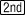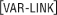after it is downloaded to the TI-89. Select "Dot" as the Mark typeUse a Zoom Data window and display the graph by pressing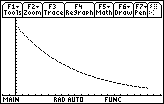The graph of the data in l1 and l2 shows a particular solution to the differential equation V' = kV and V(0) = V0. Press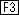to open the Trace feature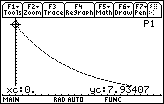The graph indicates that the initial voltage was V0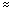7.93407 volts. Return to the Home Screen Solve the initial-value problem V' = kV and V(0) = 7.93407 deSolve(v' = k*v and v(0) = 7.93407,t,v)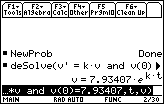Use another point on the graph to find the value of k. Return to the graph and trace to t = 30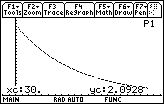The value of k can be found by solving V = 7.93407ekt with the values t = 30 and V = 2.0928. solve(v = 7.93407*e^(k*t), k) | t = 30 and v = 2.0928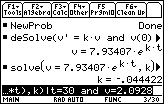Graph the function to see that the equation fits the original data well. Set y1 = 7.93407e^(-0.044422x)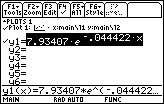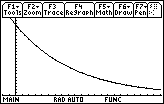The Function and the Data graphed together 19.3.1 Trace on the scatter plot of the data to t = 50 and write down the corresponding value of v. Use this value of v to find a new value for k. How well does the solution to the differential equation using this value of k fit the original data? Click here for the answer. Finding Half-Life 19.3.2 Find the half-life of the capacitor by solving the equation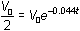for t. Click here for the answer. < Back | Next > ©Copyright 2007 All rights reserved. | Trademarks | Privacy Policy | Link Policy